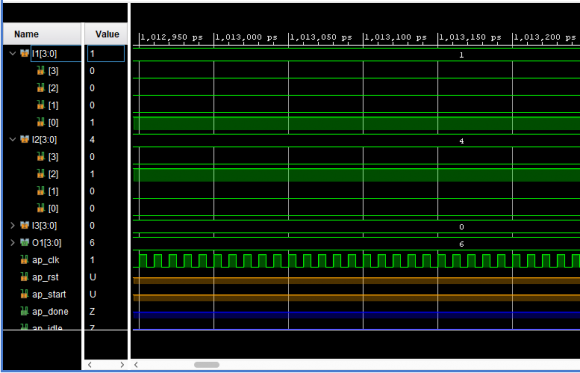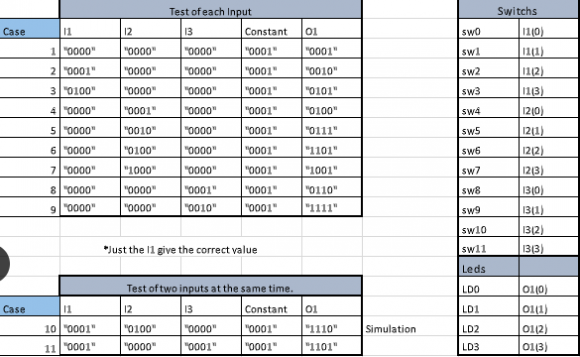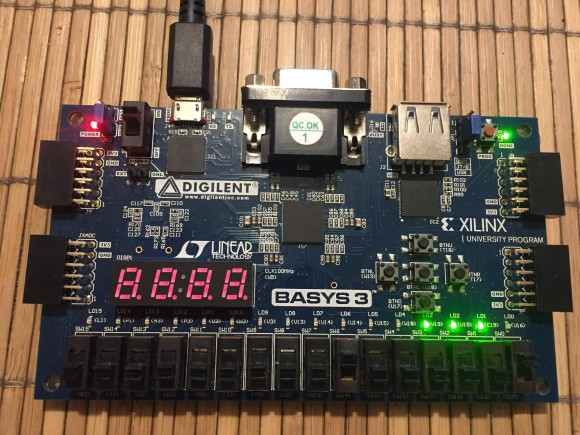## Reusing registers in VHDL FSM codeStarted by 2 years ago5 replieslatest reply 2 years ago104 views

Hello,

I need to write a Finite State Machine (FSM) in VHDL code and  want to have several computations being processed at the same time (a standard pipeline). In every state I have several operations to be calculated and I employ registers for the result of each one. I strongly need to reuse these registers, for example: Register 1 is filled in State 1 (as a result of a multiplication) and it is used in the State 2 and State 3 (as parameter of other operations), then in the State 4, I want to save a new operation result (another multiplication) in Register 1 reusing it.

My code works in Simulation in Xilinx Vivado 2019, but when I implement the desing in a real FPGA (Basys 3 Artix-7) it doesn't work. I realized that the problem is that the correct values are not saved when I reuse the registers. Sometimes, the first time I reuse them, they keep the correct value, but already in the second reuse in later FSM states, the stored values are not correct, I mean, they do not correspond to the result of the operation that I am trying to save in the register.

Next, an example of my FSM design:

LIBRARY IEEE;

USE IEEE.std_logic_1164.all;

USE IEEE.numeric_std.ALL;

ENTITY test1_arith IS

GENERIC (

ap_bit_width : positive := 4;

ap_latency : positive := 2

);

PORT (

I1 : IN STD_LOGIC_VECTOR(ap_bit_width - 1 downto 0);

I2 : IN STD_LOGIC_VECTOR(ap_bit_width - 1 downto 0);

I3 : IN STD_LOGIC_VECTOR(ap_bit_width - 1 downto 0);

O1 : OUT STD_LOGIC_VECTOR(ap_bit_width - 1 downto 0);

ap_clk : IN STD_LOGIC;

ap_rst : IN STD_LOGIC;

ap_start : IN STD_LOGIC;

ap_done : OUT STD_LOGIC;

ap_idle : OUT STD_LOGIC;

);

END;

ARCHITECTURE test1_arith_arch OF test1_arith IS

ATTRIBUTE CORE_GENERATION_INFO : STRING;

ATTRIBUTE CORE_GENERATION_INFO OF test1_arith_arch : ARCHITECTURE IS "Test,VHDLbyMOEA,{HLS_SYN_LAT=2}";

CONSTANT ap_const_logic_1 : STD_LOGIC := '1';

CONSTANT ap_const_logic_0 : STD_LOGIC := '0';

TYPE state IS (state_1,state_2,state_3);

SIGNAL state_present: state;

SIGNAL state_future: state;

SIGNAL Flag: Integer:=0;

--Signal RF : STD_LOGIC_VECTOR_array;

FUNCTION ALU ( Op: IN integer range 0 TO 23;

A, B: IN STD_LOGIC_VECTOR (ap_bit_width - 1 downto 0) )

RETURN std_logic_vector is variable Result : std_logic_vector(ap_bit_width - 1 downto 0);

variable A_int: Integer:=0;

variable B_int: Integer:=0;

variable Result_int: Integer:=0;

begin

A_int := to_integer(unsigned(A));

B_int := to_integer(unsigned(B));

With Op Select Result_int:=

to_integer(unsigned(NOT A)) When 0,

to_integer(unsigned(A AND B)) When 1,

to_integer(unsigned(A OR B)) When 2,

to_integer(unsigned(A NAND B)) When 3,

to_integer(unsigned(A NOR B)) When 4,

to_integer(unsigned(A XOR B)) When 5,

to_integer(unsigned(A XNOR B)) When 6,

(A_int + B_int) When 7,

(A_int - B_int) When 8,

(A_int * B_int) When 9,

(A_int / B_int) When 10,

ABS(A_int) When 11,

(A_int ** B_int) When 12,

(A_int MOD B_int) When 13,

to_integer(unsigned(A) & unsigned(B)) When 14,

to_integer(unsigned(A) SLL B_int) When 15,

to_integer(unsigned(A) SRL B_int) When 16,

to_integer(unsigned(A) SLA B_int) When 17,

to_integer(unsigned(A) SRA B_int) When 18,

to_integer(unsigned(A) ROL B_int) When 19,

to_integer(unsigned(A) ROR B_int) When 20,

to_integer(unsigned(A) & unsigned(B)) When 21,

to_integer(unsigned(A) & unsigned(B)) When 22,

0   When others;

return STD_LOGIC_VECTOR (TO_UNSIGNED (Result_int, (ap_bit_width)));

END FUNCTION;

SHARED VARIABLE R1:std_logic_vector(ap_bit_width - 1 downto 0);

BEGIN

OP_FSM : PROCESS (state_present)

BEGIN

CASE state_present IS

WHEN state_1=>

R1 := ALU(Op => 7 ,A => I1,B => I2);

Flag<=1;

IF (Flag=1) THEN

state_future <= state_2;

END IF;

WHEN state_2=>

R1:= ALU(Op => 7 ,A => R1, B => I3);

Flag<=2;

IF (Flag=2) THEN

state_future <= state_3;

END IF;

WHEN state_3=>

O1<= ALU(Op => 7 ,A => R1,B => "0001");

Flag<=3;

IF (Flag=3) THEN

state_future <= state_1;

END IF;

END CASE;

END PROCESS OP_FSM;

CLK_FSM : PROCESS (ap_clk)

BEGIN

IF (ap_clk = '1' AND ap_clk'EVENT) THEN

state_present <= state_future;

END IF;

END PROCESS CLK_FSM;

END test1_arith_arch;

In this case, I want to reuse R1 and it works well in Simulation with Xilinx Vivado (1 + 4 + 0 + 1 = 6):Unfortunately, in the Basys 3 FPGA Artix-7 I don't get the correct results:In this figure, I show the Case 10 in a FPGA, it should get 6 (1 + 4 + 0 + 1) as result, but it gets 14 instead:In the tests that I have been doing I realized that it works better when before assigning a new value in the registry the value of the record is made zero before reassigning a value, for example:

WHEN state_3=>

R4<="0000"

IF( R4 = "0000") then

R4<= ALU(Op => 7 ,A=> R2,B=> R3, C =>"0000");

Flag <=3;

IF (Flag =3) THEN

state_future <= state_4;

END IF;

END IF;

Using this form I can reuse a register once, the second time I want to reassign a value to the register, incorrect values are shown in the output.

I declarated the registers as SHARED VARIABLE and SIGNALS and I have the same problem with both.

I appreciate any suggestion or idea, thanks a lot.

[ - ]I believe R1 is in trouble as your states stay for more than one clock due to flag assignment then state transition.

[ - ]Now I see your flag signal is not in clocked process so my first post needs correction but I see you have combinatorial feedback on R1

[ - ]Thank you so much. How can I fix my FSM to achieve that it works in the FPGA device?

[ - ]